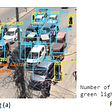# Multi-class Classification of Mathematical-Numbers | DNN+dropout | Part2

In case you are directly landing here, it’s highly recommended to have a view at this story.

In this blog, we shall be using KERAs framework with TensorFlow backend, in order to create a Deep Neural Network and would apply some drop-out. We shall be using MNSIT dataset, which consists of 28*28 grayscale images, representing the hand-written images of numeric digits 0 to 9. The dataset is partitioned in 2 parts :-

• Training dataset of 60,000 images.
• Testing dataset of 10,000 images.

This is a classic example of multi-class-classification, where we would be classifying, the input image into 1 amongst 10 classes of decimal-digits (0 to 9). Our Deep-Neural-Network have 2 hidden layers, in addition to the input & output layer, like the way we have demonstrated below :-

We shall be implementing the drop-out in both of the hidden layers.

First, we import important libraries that we shall be using throughout our demonstration. We shall be using the tensorFlow V1 throughout the setup, therefore we explicitly also disable the TF V2 behaviour.

Next, we initialise the random-number-generator & set the seed so that, we can keep on using the same set of instances or random-numbers in every run of the program.

We would be using the MNIST hand-written data-set, which is already provided as a built-in data-set in the KERAs framework. We, therefore first create the instance of MNIST dataset.

Next, we would be loading the data (from MNSIT data-set) into the current environment.

Let’s first understand the meaning of the 4 variables created above :- The training set is a subset of the data set used to train a model.

• `Xtrain` is the training data set.
• `Ytrain` is the set of labels to all the data in `Xtrain`.

The test set is a subset of our entire data-set, that we shall be using to test our model, after the model has gone through initial vetting by the validation set.

• `Xtest` is the test data set.
• `Ytest` is the set of labels to all the data in `Xtest`.

Coming back on the MNSIT dataset, we have following configuration :-

• Training dataset of 60,000 images, stored in Xtrain.
• Testing dataset of 10,000 images, stored in Xtest.

Also, please note that, this is set of hand-written numbers, stored as gray-scale-images. Each image is being stored as the matrix of size, 28 * 28 with pixel values taking a value from in range (0 to 255). Below is any sample image (from this MNSIT dataset) :-

Understand that, each image is of size 28 * 28. Each pixel can be varying within range of (0, 255). Each value represents some density of color. Note that, each image is a 2d array of size (28 * 28). Let’s go ahead and see, what’s the pixels value 59,999th image are :-

Now, let’s go ahead and see the label for this particular image, as given in the MNSIT test dataset :-

Similarly, as we have also learnt that, there are only 10,000 images into the test-data-set.

Now, let’s go ahead and see the label for this particular image, as given in the MNSIT test dataset :-

Data-Pre-Processing :- Next, we need to perform the pre-processing on the images.

• The input images are converted into a tensor of size (28 * 28), using the “reshape()” function.
• Then, each image is normalised, in order to convert the pixel values from range (0 to 255) to range (0 to 1). Also, we shall be converting the values into float type values.

Remember from our previous screenshots above that, each image was originally a 2d matrix of size 28*28. Let’s now go ahead and convert the input image from 2d into 1d of size: 784 * 1, using the “reshape()” function. Note that, after this reshape operation, each image is a 1d array of size 784. Also note that, post this operation, we can neither visually see the image anymore nor plot it using pyplot.

Next, let’s perform the Normalisation operation. Note that, initially each pixel in the image has gotten the value from range (0 to 255). Post the normalisation operation, value of the pixel would be ranging from (0 to 1).

Therefore, each pixel value would now become of float type :-

Next, let’s also perform the similar operations on the Xtest dataset :-

• Reshape → We shall be reshaping each image in the test dataset from 2d (each image of size 28 * 28) into 1d (each image of size 784).
• Normalisation → We then perform normalisation where each pixel value is converted from range of (0 to 255) to the range of (0 to 1).

Note that, the output values into the data-set are categorical in nature with values in range of (0 to 9). Therefore, this categorical data is first converted into vector using One-Hot-Encoding approach :-

Keras library provides us out-of-the-box method to “to_categorical”.

Above step completes the pre-processing step for both input images and output categories. Revision to note that :-

• Xtrain contains the 60,000 images, which we shall be using for training.
• Ytrain contains the corresponding labels for 60,000 images in Xtrain dataset.
• Xtest contains the 10,000 images, which we shall be using for testing/validation.
• Ytest contains the corresponding labels for 10,000 images in Xtest dataset.

Let’s now build the simplest & sequential DNN using Keras. We are planning to use the 4 layers in this Neural Network.

First Layer is an input layer, which expects input-tensor of size 784 (i.e. each input image to our model is of size 784*1). Recall that, earlier above, we had converted(reshaped) all of our input images from 2d to 1d.

Note that, only the input layer specifies the input_size. The input-layer (i.e. First layer) is defined as dense-layer with 50 neurons and ‘relu’ activation function. Dense-Layer implies that, all neurons of one layer are connected to all neurons of next layer.

Second Layer is defined as a hidden layer with 60 neurons and ‘relu’ activation function. This is also a dense-layer, but we added a drop-out of 50%. This implies that, a total of 50% of neurons in the 2nd layer would be Active at any moment of time.

Question: What is DropOut actually ?

Question: What is the purpose of adding DropOut ?

Question: What does OverFitting stands for ?

Question: On which dataset is DropOut applied usually ?

Question: How to visualise the neuron with drop-out ?

Question: Has there been research / studies, which demonstrates the improvements brought-in by Drop-Out ?

Coming back to the Third Layer Is again defined as a hidden layer with 30 neurons and ‘relu’ activation function. This is also a dense-layer, but here also we have added a drop-out of 50%.

Fourth Layer is the last layer, which is again defined as the output layer with 10 neurons(note that, each neuron represents a specific category) and it uses ‘softmax’ activation function, in order to perform classification accurately.

Summary of the Model Here is how our entire model looks like :-

Note that, we have in-total of 44,450 parameters, in order to be trained.

Configuring Model :- Let’s now configure our recently created DNN model for training.

• We shall be defining “Categorical Entropy” as the Cost-Function / Loss-Function.
• The metrics used for evaluating the model is “accuracy”.
• We are planning to use ADAM optimiser on the top of SGD (Standard Gradient Descent), in order to minimise the cost-function more robustly.

Once the configuration of our model is complete, we can proceed for Training.

Training of Model :- Given the enormous(60K) data-set that we have got, we shall not be using “Full-Batch-Gradient-Descent” because of expensive computation being involved there, therefore we are planning to use “Mini-Batch-Gradient-Descent” approach, in order to minimise the LOSS :-

Let’s understand few things from above step :-

• Epoch :- One Epoch is when an ENTIRE dataset is passed forward and backward through the neural network only ONCE. We usually need many epochs, in order to arrive at a optimal learning curve.
• Batch size: Since we have limited memory, probably we shall not be able to process the entire training instances(i.e. 60,000 instances) all at one forward pass. So, what is commonly done is splitting up training instances into subsets (i.e., batches), performing one pass over the selected subset (i.e., batch), and then optimising the network through back-propagation.
• Validation Split: It means that out of total dataset, 2% of data is set aside as the cross-validation-set.

As training of the DNN model progresses, both the (accuracy and loss) are stored as a list in the object. Final (Training loss and Loss) can be obtained from the history object.

• The last element of the history object (model_fit.history[‘loss’])gives us the final loss after training process.
• The last element of the history object (model_fit.history[‘acc’])gives us the final accuracy after training process.

Next, We can also plot the graph for Validation-Accuracy. Below graph signifies that : As the no. of epochs progresses, accuracy also increases.

Next, We can also plot the graph for Validation-Loss. Below graph signifies that : As the no. of epochs progresses, loss-value also decreases.

Next, We can evaluate our thus build model with the help of testing dataset of size 10,000. The “evaluate” function gives us the testing accuracy and testing loss as the output.

From above snapshot, we can observe that, our DNN model with DropOut, is giving the testing accuracy of 96.86%. We can try to improvise(i.e. tune) this model’s accuracy by playing on following parameters :-

• Changing the number of hidden layers itself. (Note that, we have used 4 layers in total in aforesaid demonstration).
• Changing the number of neurons in each of the involved layers (input/dense/output layer).
• By playing on the value of the eTa i.e. Learning-Rate.
• Choice of Activation functions at each layer.
• By modifying the various optimisers like RMSProp, etc.

Thanks for reading through this and we shall meet you in another article.

--

--

--

Software Engineer for Big Data distributed systems

Love podcasts or audiobooks? Learn on the go with our new app.

## Fast and Arbitrary Style Transfer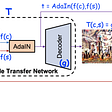## Implementation of RNN’s in Text Classification Tasks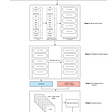## Can Machine Learning Tell Us How Smart You Are?## Using TFRecords to Train a CNN on MNIST## Predictions in 30 mins using new Cloud Pak for Watson AIOps## Relighting and Material Editing with Implicit Representations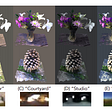## Can We Decode FMRI datasets using Neural Networks? (Arguably for Ailurophiles)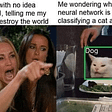## Global error minimization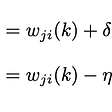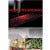Software Engineer for Big Data distributed systems

## Solar panel defect detection using Vision Intelligence Systems | ignitarium.com## Top 5 Classification Algorithms in Machine Learning## Smart Traffic Management System